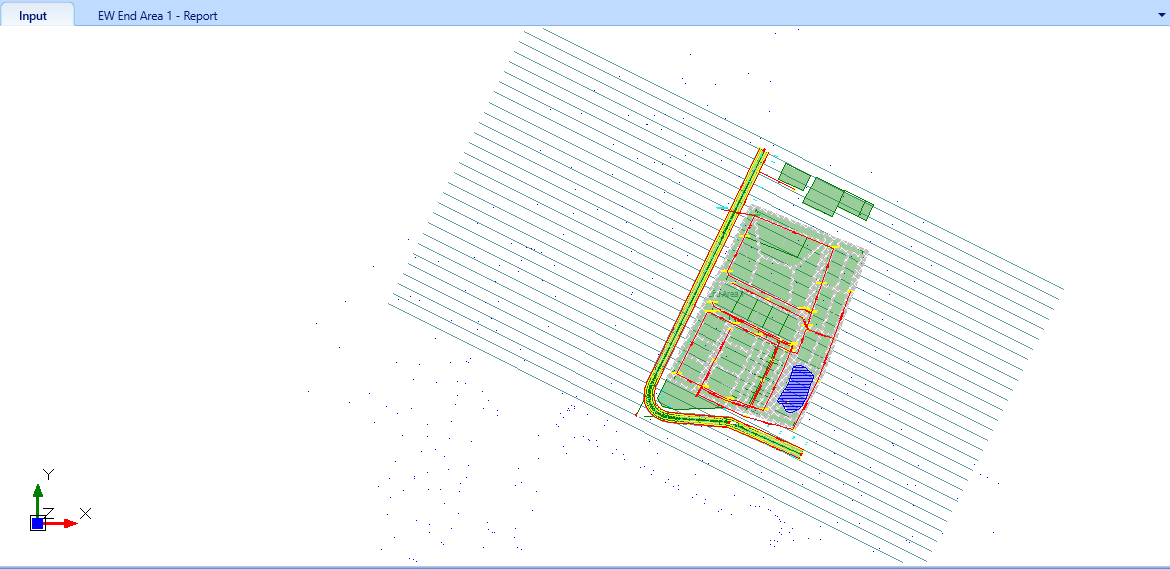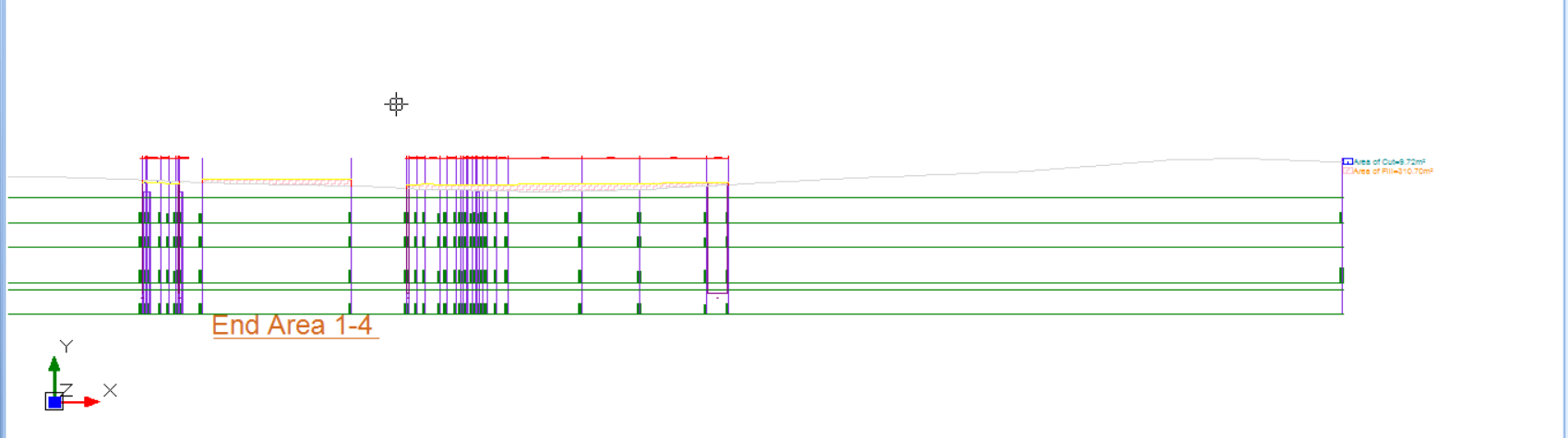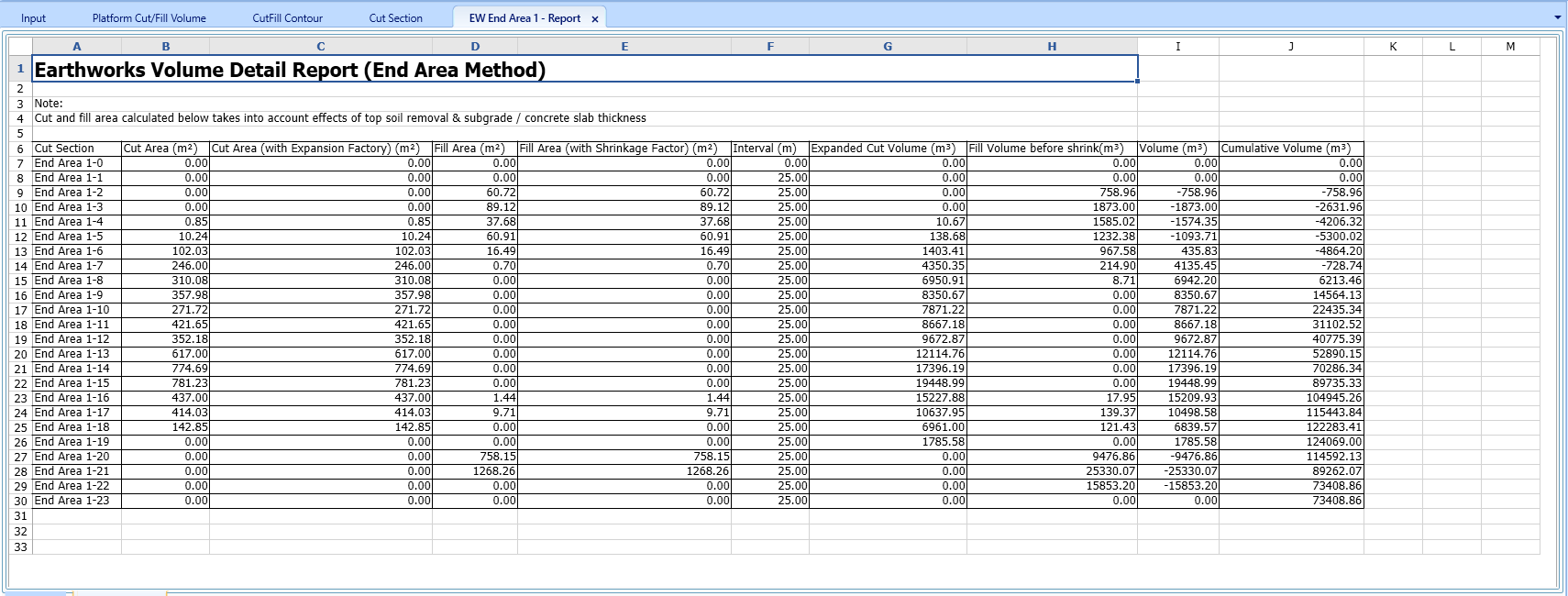603 - 5885 1250 (4 lines) info@mes100.com
Select Page

# Calculation of End Area Method in Earthwork

End Area Method, also known as Average End Areas method, is a popular method to estimate the earthwork volume. Like grid method, it is also easy to use, easy to understand and can be carried out by someone even with minimum mathematical training.

The procedure to compute cutfill volume from End Area Method is simple.

## The Procedure#

1. First define cut sections, separated by a fix interval, say 25 m. Make sure that your cut sections adequately cover your project area in both latitude and longitude direction, as shown below:2. For each cut section, calculate the respective cut and fill area. You can do this by measuring the area of the cut and fill via AUTOCAD command.3. Compute each section volume by using the formula $V_i=\frac{(A_{i-1}+A_i)L}{2}$, where $L$ is the end area interval.
1. Tabulate the cut section area, and then sum up the volume for both cut and fill in an excel spreadsheet, then you can already get your end area cutfill volume!## Discussion#

1. Although End Area Method is a popular cutfill method, but it is by no means the most accurate method. This title goes to DTM ( Digital terrain method)
2. The reason being, like grid method, it depends on the cut section interval. You will have to use extremely small interval to capture accurate earthwork results, if your cross section varies a lot over short interval of space.
3. Also, the cut section is generated from the interpolation of the ground level, which itself is an approximation. So when you are taking the volume as a function of the two subsequent cut section areas, then it is a double approximation, which further reduces the accuracies.